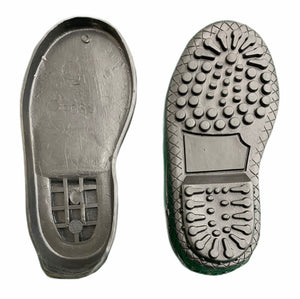# Shoe Sole - Ross

• R 12.50
Unit price per
Tax included.

Ross Shoe soles

Available in kids sizes 21 to 33

Sold in pairs. Prices may differ according to sizes.

Size 21 = 4
Size 22 = 5
Size 23 = 6
Size 24 = 7
Size 25 = 8

Size 26 = 9
Size 27 = 10
Size 28 = 11
Size 29 = 12

Size 30 = 13
Size 31 = 1
Size 32 = 2
Size 33 = 2.5